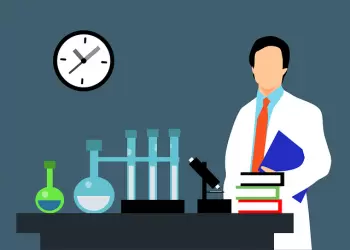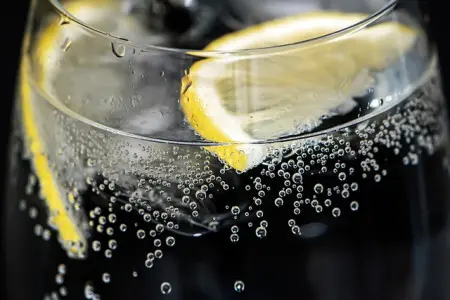# Gas Laws: List and DescriptionThe gas laws are a set of physical-chemical laws that describe the behavior of gases when they are in a closed system.

These laws were developed at the end of the 18th century and mathematically relate the pressure, temperature and volume for a given gas.

The equation of state of an ideal gas is derived from the kinetic theory of gases, which explains the behavior of gas particles (atoms and molecules). The moving particles (kinetic energy) collide with each other and with the walls of the container, influencing the pressure, temperature and volume.

The most important gas laws are:

## Ideal Gas Law

The ideal gas law is one of the gas laws and represents the equation of state of an ideal gas. It is a good approximation of the behavior of some gases under some conditions, although it has some limitations.

The ideal gas law can be expressed with the following equation:

P·V=n·R·T

Where,

• P is the pressure.

• V is the volume.

• n is the amount of the substance or number of moles.

• R is a constant that depends on the gas.

• T is the temperature.

## Boyle-mariotte Law

The Boyle-Mariotte law states that at constant temperature, the volume of a gas is inversely proportional to the increase in pressure.

This law describes the pressure-volume relationship of a gas in an isothermal process, that is, without changing the temperature. From the point of view of modern physics, the law is a consequence of the Clapeyron-Mendeleev equation.

Robert Boyle established this law experimentally in 1662. In turn, Edm Mariotte rediscovered it on his own in 1676.

## Gay-lussac's Law

Gay-Lussac's law states that at constant volume, the pressure of a given mass of gas is directly proportional to its absolute temperature. That is, as the temperature increases, the pressure increases if the volume remains constant.

P/T = constant

Gay-Lussac's law is also known as the law of isochoric transformation because it is carried out at constant volume. It was discovered by the French physicist Jacques Charles in 1787 but was first stated by Louis Joseph Gay-Lussac in 1802.

Jacques Charles demonstrated that when a gas undergoes an isochoric transformation, the relationship between its pressure and temperature remains constant.

The molecular explanation of this law is that by raising the temperature of a quantity of gas, the average speed of its molecules increases. The molecules collide more frequently with the walls of the container and have a stronger impact, thus increasing the pressure exerted by the gas molecules on them.

In this site we will use the name Gay-Lussac's law to refer to the relationship between pressure and temperature at constant volume and Charles's law for the relationship between volume and temperature at constant pressure.

## Charles's Law

Charles's law states that if the pressure and quantity of a gas are unchanged, the relationship between volume and temperature remains constant and are directly proportional to each other.

V/T = constant

Sometimes the name Gay-Lussac is also used to refer to Charles's law that relates the temperature and pressure of a gas at constant pressure. In fact, Louis Joseph Gay-Lussac announced Charles's law in 1802, while Jacques Charles had discovered it 15 years earlier, in 1787.

Avogadro's law states that gases with the same volume, temperature, and pressure contain the same number of particles (or molecules). So the number of molecules in a specific volume of gas is independent of the size or mass of the gas molecules.

The number of molecules in one mole of substance is Avogadro's number: approximately 6.022·10 23 particles/mol.

Consequently, the densities of the different gases are, under the same conditions of temperature and pressure, proportional to their molecular masses.

This law is named after Amedeo Avogadro, who proposed it in 1811.

## Henry's LawHenry's law, formulated by William Henry in the 19th century, is a fundamental principle that establishes a relationship between the concentration of a gas in a solution and its partial pressure in the gas phase above the solution.

Although it has limitations under extreme conditions, this law is widely used in chemistry, biology, chemical engineering, and geology to understand and manipulate the solubility of gases in liquids.

## Graham's Law

Graham's law, proposed by British chemist Thomas Graham, states that the rate of diffusion (or effusion) of gas molecules is inversely proportional to the square root of their density.

In other words, lighter gases diffuse or escape more rapidly than heavier gases through a porous medium or through small openings.

For example, if you compare the diffusion rates of helium (a light gas) and xenon (a heavier gas) through a rubber balloon, helium will diffuse much faster due to its lower density.

## Dalton's Law (law of Partial Pressures)

Dalton's law, proposed by British chemist John Dalton, states that the total pressure exerted by a mixture of gases is equal to the sum of the partial pressures of each gas in the mixture. In other words, each gas in a mixture exerts pressure as if it occupied all the space alone, without interacting with other gases present.

For example, if you have a gas mixture composed of oxygen and nitrogen, the total pressure exerted by the mixture is the sum of the partial pressures of oxygen and nitrogen. This law is fundamental in gas chemistry and is used in determining the composition of gas mixtures.

Author:

Published: December 1, 2021
Last review: September 27, 2023